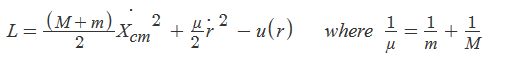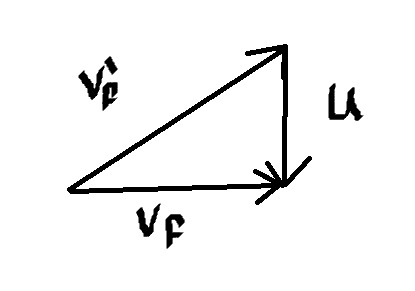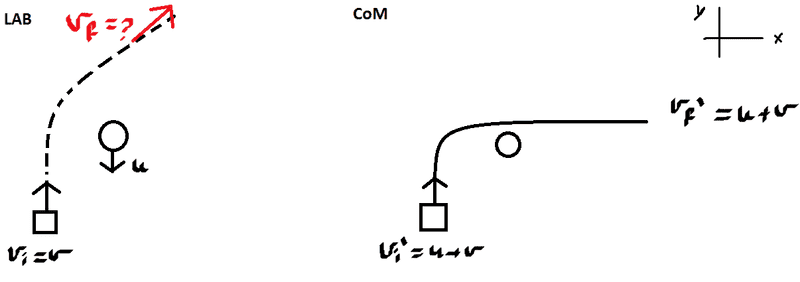# Gravitational slingshot

MMS

## Homework Statement

Relative to space, a spacecraft moving at velocity v in the y direction (y is upwards, x to the right) is getting closer to a planet moving at velocity u in the opposite y direction. The spacecraft does a hyperbolic trajectory with eccentricity sqrt(2) so that it ends up moving in the x direction.
When the spacecraft is far away from the planet, what is its relative speed to space?

## Homework Equations

Conservation of momentum
Conservation of energy

## The Attempt at a Solution

.[/B]
We got this problem in my analytical mechanics class.

Anyway, the Lagrangian for the two body problem is (taking M is the mass of the planet and m is the mass of the spacecraft):There isn't any dependency of the generalized coordinate Xcm or time, therefore, according to noether's theorem, there is conservation of energy and momentum.
At this point, I assumed, that since the mass of the planet is much larger than the mass of the planet, mu=m. Also, the change in the magnitude of the velocity of the planet is negligible.
Assuming that the planet deflected in some angle theta (since the spacecraft couldn't have simply gathered momentum in the x direction), I got, from conservation of momentum in the x direction:

v'=(M/m)[cos(theta)]u

and in y:

mv=Mu[1-cos(theta)]

but I don't know the masses or the angle theta..

From conservation of energy, with my assumptions, I simply get that the magnitude of v before and after is the same.

Not sure if my approach is right or it is supposed to be dependent of the masses or angle like I've written down or I missed something so I'd love some feedback and help with it.

Thanks and happy holidays.

Staff Emeritus
Homework Helper
Gold Member
2021 Award
First of all: There is no such thing as motion relative to space, so the problem is very badly formulated. Objects can move relative to each other and be in inertial frames or not, but there is no such thing as motion relative to space.

To uour questions: Just as you cannot simply neglect the planet when doing momentum conservation, you cannot neglect it when doing energy conservation either. It is therefore not correct to assume that the spacecraft will end up with the same speed.

MMS
First of all: There is no such thing as motion relative to space, so the problem is very badly formulated. Objects can move relative to each other and be in inertial frames or not, but there is no such thing as motion relative to space.

To uour questions: Just as you cannot simply neglect the planet when doing momentum conservation, you cannot neglect it when doing energy conservation either. It is therefore not correct to assume that the spacecraft will end up with the same speed.

First off, thanks for the reply.

As for the way the problem is formulated, I simply quoted it but I do understand relative motion between bodies.

As for the problem itself, I didn't neglect the planet. I meant that the magnitude doesn't change (approximately).
Rather, it deflects in some angle theta and therefore its velocity changes in the x and y direction.

Staff Emeritus
Homework Helper
Gold Member
2021 Award
As for the problem itself, I didn't neglect the planet. I meant that the magnitude doesn't change (approximately).
Rather, it deflects in some angle theta and therefore its velocity changes in the x and y direction.

But the magnitude does change. This was the point of my post. Compare this to an elastic collision between a light and heavy body seen in a frame where the heavy object is moving. In this frame, the kinetic energy of the light object definitely will change.

Hint: In the center of mass frame, which is approximately the rest frame of the planet, you can use your argument.

MMS
But the magnitude does change. This was the point of my post. Compare this to an elastic collision between a light and heavy body seen in a frame where the heavy object is moving. In this frame, the kinetic energy of the light object definitely will change.

Hint: In the center of mass frame, which is approximately the rest frame of the planet, you can use your argument.

I think I got it. I'll update with a solution later on.

Thanks!

P.S: Just to be sure, is there a different method\approach to these types of problems?
I mean, I got this question in my analytical mechanics but I'm using basic methods from classical mechanics..

MMS
Okay I didn't get anywhere with what I have.
I believe what you wanted to convey is reducing the two body problem into one and using the relative speed u+v as the speed of the spacecraft. The problem is I got nowhere with this. I substituted this into the Lagrangian but I'm missing the potential energy which isn't given.
Any other hints?

Last edited:
Staff Emeritus
Homework Helper
Gold Member
2021 Award
Show your working and explain why it gets you nowhere.

MMS
I've basically written down anything I could to try and relate one thing to another in analytical mechanics since I couldn't figure out how to deal with it in classical mechanics (missing the masses and deflection angle).
Took the Lagrangian (the one I wrote above) and wrote the E-L equations for r (the relative coordinate between the two and it kills the Xcm part as well ) ignoring the planet as it is my rest frame and then I'm left with a body moving at speed u+v
Written down the canonical momentum for r with r\dot=u+v, the angular momentum and the energy. I tried doing something with the Runge-Lenz vector as well. However, despite all this, I'm missing some information to proceed with my solution (for example the relative distance between the two, the masses and the potential energy).

Any help on where I could at least start or what to do? I feel like I'm making this problem more complicated than it actually is...

Thanks!

MMS
Anyone?

Staff Emeritus
Homework Helper
Gold Member
2021 Award
In the CoM frame, you do not need to know anything about the relative distance or the actual masses. The information that the kinetic energy of the spaceship in the CoM frame is conserved is sufficient. The space ship is moving with velocity u+v in this frame. What does this tell you about the velocity in the lab frame? What do you know about the velocity in the lab frame?

MMS
Alright, here's a new attempt at solution using your guidance. Please, take a look at it:

v' denotes in the CoM
v denotes in the lab.

From the CoM frame we have the velocity of the spacecraft as v'_i=u+v (VECTORS)
The energy of the spacecraft in the rest frame is conserved therefore at infinity it will have the same velocity but in a different direction. Hence, the magnitude |v'|=|v'_f|=u+v.
Since the spacecraft couldn't have simply gained momentum in a different direction and is affected by the planet, I assumed the v'_f=(u+v) to be at some direction w.
Going back to the lab frame of reference by adding up u, we know that the velocity v_f is in the x direction. therefore v_f=v'_f+u
Using the Pythagorean theorem I get that v_f=sqrt[(v^2)+(2uv)].
I'll add up a sketch in a second of the vectors.If I'm wrong anywhere, please, it would help if you could denote where , why and how I could get it right because I must hand this in tomorrow.

Thank you!

Last edited:
MMS
Also anyone else is welcome to check out my attempt.

MMS
CORRECTION:
Apparently (according to the teacher in our class) the eccentricity is given in the planet's rest frame. Hence, it does the hyperbolic trajectory there and not relative to "space". Which makes my answer wrong. I'll correct it and update in a second.

MMS
This, I believe, is the right sketch:MMS
Again, the same explanation as above for the velocities and all.
I believe that the final velocity v_f is now (as a vector) : v_f=(u+v,-u)
Therefore its magnitude is |v_f|=sqrt[(2u^2)+(v^2)+(2uv)] .

What do you think Orodruin?

Mentor
Edit: Ah, it moves in x-direction as seen by the planet? Then the new answer is correct.

MMS
Looks right.

Which is right? the explanation or the final answer?
Because I corrected the final answer in my last post.

Mentor
See my edit above, I didn't see your edit before I posted the old version of my post.

MMS
Edit: Ah, it moves in x-direction as seen by the planet? Then the new answer is correct.

He sent a mail saying that the hyperbolic trajectory is seen from the planet's frame of reference and that relative to "space" it isn't hyperbolic. Hence, it changes its motion to x^^ in the planet's frame of reference.

What do you think?

Mentor
Then the new answer is correct.
This.

MMS
This.
Okay, thank you and merry christmas!

MMS
More thoughts on my solution and answer are much welcome though.